# Spatial relationships

GeoAnalytics Engine offers various tools and functions that enable you to analyze and understand the spatial relationships between geometries. For example, to find the number of homes that were affected by a recent flood, you could join the homes to the flood boundary using the Within spatial relationship.

## Using functions to analyze spatial relationships

Functions analyze spatial relationships using geometry columns from a DataFrame. Depending on the function, the input can be one or two geometry columns, and in some cases additional spatial considerations (for example, a distance or boundary).

Functions return values of `True` or `False` for each geometry depending on whether or not it meets the spatial conditions specified.

## Using tools to analyze spatial relationships

Tools analyze spatial relationships using DataFrames as input. Each input DataFrame must have only one geometry column set in order to be used in an analysis tool.

Tools return DataFrames that contain geometries and columns that can be used to understand the spatial relationship. The contents of the output DataFrame depend on the tool that was used. Some results contain all input geometries with new columns indicating the relationship, and other results contain only the geometries that met the specified condition. See the respective tool help for more information.

This topic outlines spatial relationships available in the tools Spatiotemporal Join and Group By Proximity.

## Spatial relationships between geometries

The following legend applies to all the examples in this topic:

• Geometry 1—Blue

• Geometry 2—Yellow

• Geometry 1 exhibits the selected spatial relationship with geometry 2—Red highlight

In a spatial relationship, each type of geometry (point, polyline, and polygon) has an interior and a boundary. How the interiors and boundaries of two geometries compare determines the spatial relationship they exhibit. The following image outlines the geometries, boundaries, and interiors of points, polylines, and polygons:

### Geometry types and supported relationships

The following table lists the supported spatial relationships between geometries of a given type (for example, the relationship of points to other points):

### Equals

Geometry 1 is equal to geometry 2 if their interiors are identical and the geometry types are the same.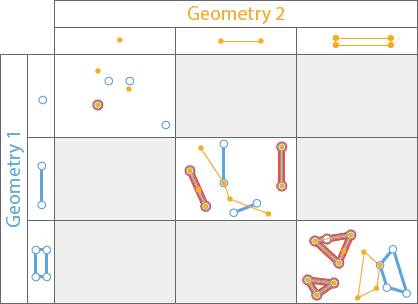The blue geometries from geometry 1 are highlighted with red when they are equal to the yellow geometries from geometry 2.

You can access this spatial relationship in the following ways:

• Use the Spatiotemporal Join tool with a value of `Equals` for the `spatial_relationship` parameter. Geometries in red will be joined.
• Use the spatial function ST_Equals. Geometries in red will return a value of `True`.

### Intersects

Geometry 1 intersects geometry 2 if it shares any portion of its geometry with geometry 2. If geometry 1 contains, is within, crosses, touches, or overlaps geometry 2, it intersects.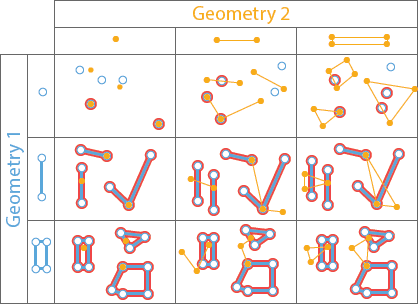Geometry 1 in blue is highlighted with red when they intersect the yellow geometry 2.

You can access this spatial relationship in the following ways:

• Use the Spatiotemporal Join tool with a value of `Intersects` for the `spatial_relationship` parameter. Geometries in red will be joined.
• Use the Group By Proximity tool with a value of `Intersects` for the `spatial_relationship` parameter. Geometries in red will be grouped with the intersecting geometries.
• Use the spatial function ST_Intersects. Geometries in red will return a value of `True`.

### Contains

Geometry 1 contains geometry 2 if geometry 2 is a subset of geometry 1, with intersecting interiors. If geometry 2 is on the boundary and intersects the interior, then geometry 1 contains geometry 2. If geometry 2 is only on the boundary (and not the interior), then geometry 1 does not contain geometry 2. This is the inverse of the Within function operator.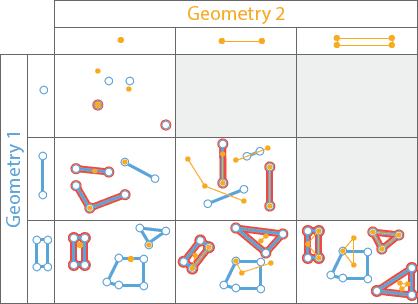Geometry 1 in blue is highlighted with red when they contain the yellow geometry 2.

You can access this spatial relationship in the following ways:

• Use the Spatiotemporal Join tool with a value of `Contains` for the `spatial_relationship` parameter. Geometries in red will be joined.
• Use the spatial function ST_Contains. Geometries in red will return a value of `True`.

### Within

Geometry 1 is within geometry 2 if it is completely inside geometry 2. A geometry is within if the intersection of the interiors is nonempty and geometry 1 is a subset of geometry 2. If there is no intersecting interior, then geometry 1 is not within geometry 2. This is the inverse of the Contains operator.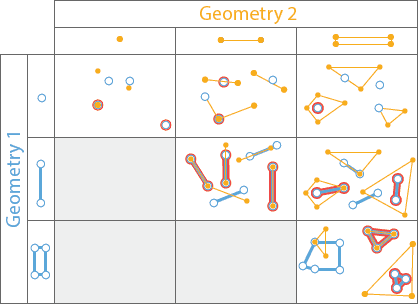Geometry 1 in blue is highlighted with red when they are within the yellow geometry 2.

You can access this spatial relationship in the following ways:

• Use the Spatiotemporal Join tool with a value of `Within` for the `spatial_relationship` parameter. Geometries in red will be joined.
• Use the spatial function ST_Within. Geometries in red will return a value of `True`.

### Crosses

Two polylines cross if their intersection contains only points, and at least one of the points of intersection is internal to both polylines. A polyline and polygon cross if a connected part of the polyline is partly inside and partly outside the polygon. A polyline and polygon cross if they share a polyline in common on the interior of the polygon, which is not equal to the entire polyline. Geometry 1 and geometry 2 must be either polylines or polygons.

You can access this spatial relationship in the following ways:

• Use the Spatiotemporal Join tool with a value of `Crosses` for the `spatial_relationship` parameter. Geometries in red will be joined.
• Use the spatial function ST_Crosses. Geometries in red will return a value of `True`.

### Touches

Geometry 1 touches geometry 2 if they have an intersecting vertex, but the geometries do not overlap.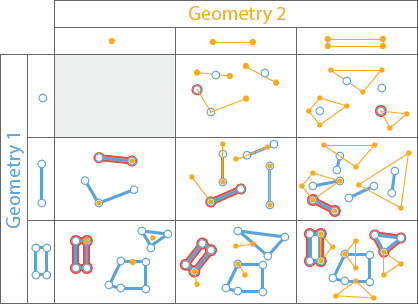Geometry 1 in blue is highlighted with red when they touche the yellow geometry 2.

You can access this spatial relationship in the following ways:

• Use the Spatiotemporal Join tool with a value of `Touches` for the `spatial_relationship` parameter. Geometries in red will be joined.
• Use the Group By Proximity tool with a value of `Touches` for the `spatial_relationship` parameter. Geometries in red will be grouped with the touching geometries.
• Use the spatial function ST_Touches. Geometries in red will return a value of `True`.

### Overlaps

Two geometries overlap if they are the same geometry type and their intersection also has the same geometry type, but the geometry that intersects is different from both of the original geometries.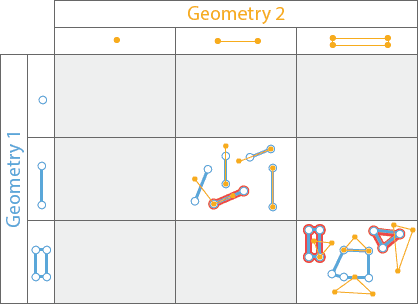Geometry 1 in blue is highligted in red when it overlaps geometry 2.

You can access this spatial relationship in the following ways:

• Use the Spatiotemporal Join tool with a value of `Overlaps` for the `spatial_relationship` parameter. Geometries in red will be joined.
• Use the spatial function ST_Overlaps. Geometries in red will return a value of `True`.

### Planar Near and Geodesic Near

Conceptually, these operators buffer geometry 1 using the specified distance and return all the geometries from geometry 1 that intersect geometries in geometry 2 with the buffer applied. Geodesic Near is the same as Planar Near, except geodesic distance is used rather than planar distance. Choose the geodesic operator if your data covers a large geographic extent or the coordinate system of the inputs is geographic.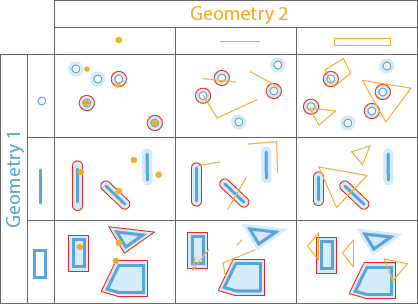Geometry 1 in blue, with a near distance buffer (light blue), is highlighted with red when they are near the yellow geometry 2.

The Planar Near and Geodesic Near function works similar to the DWithin function.

You can access this spatial relationship in the following ways:

• Use the Spatiotemporal Join tool with a value of `NearPlanar` or `NearGeodesic` for the `spatial_relationship` parameter. Geometries in red will be joined.
• Use the Group By Proximity tool with a value of `NearPlanar` or `NearGeodesic` for the `spatial_relationship` parameter. Geometries in red will be grouped with the near geometries.
• Use the spatial function ST_DWithin.

### Disjoint

Disjoint takes two geometry columns as input and determines whether they are intersecting or not. If geometry 1 does not intersect geometry 2 they are disjoint and a value of `True` is returned; otherwise a value of `False` is returned.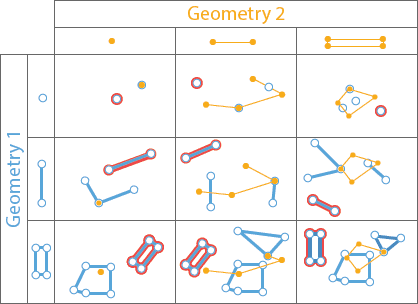Geometry 1 in blue is highligted in red when it is disjoint from geometry 2.

You can access this spatial relationship using the spatial function ST_Disjoint.

### EnvIntersects

EnvIntersects takes a geometry column and envelope values (xmin, ymin, xmax, ymax) as input. If the geometry intersects with the envelope a value of `True` will be returned; otherwise a value of `False` is returned.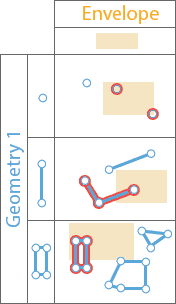Geometry 1 in blue is highlighted in red when it intersects the envelope in yellow.

You can access this spatial relationship using the spatial function ST_EnvIntersects.

### Relate

Relate takes two geometry columns as input and considers a DE-9IM pattern matrix to determine the relationships between each pair of geometries. If the relationship between geometry 1 and geometry 2 meet the conditions specified by the DE-9IM matrix a value of `True` will be returned; otherwise a value of `False` is returned.

You can access this spatial relationship using the function ST_Relate.

### DWithin

See Near to learn about DWithin.